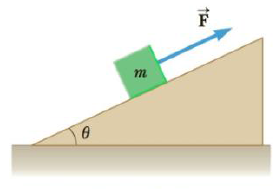Chapter 4, Problem 24P

Chapter
Section
Textbook Problem

A block of mass m = 5.8 kg is pulled up a θ = 25° incline as in Figure P4.24 with a force of magnitude F = 32 N. (a) Find the acceleration of the bloc k if the incline is frictionless. (b) Find the acceleration of the block if the coefficient of kinetic friction between the block and incline is 0.10.Figure P4.24

(a)

To determine
The acceleration of the block.

Explanation

Given Info: Mass of the block is 5.8 kg. Magnitude of the force is 32 N. Angle of inclination is 25ο .

The free body diagram is given below.

From the above diagram,

Fmgsin25ο=ma                              (I)

• F is the pulling force.
• N is the normal force.
• g is the acceleration due to gravity.
• a is the acceleration.
• m is  the mass of the block.

On re-arranging Equation (I),

a=Fmgsin25ο (II)

Substitute 32 N for F, 9.8ms2 for g and 5

(b)

To determine
The acceleration of the block when there is friction.

Still sussing out bartleby?

Check out a sample textbook solution.

See a sample solution

The Solution to Your Study Problems

Bartleby provides explanations to thousands of textbook problems written by our experts, many with advanced degrees!

Get Started

Find more solutions based on key concepts